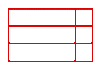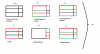BrainDen.com - Brain Teasers

## Question

While flying over farmland, a pilot notices the rectangular shape of the fields below. She sketches the lines that divide the fields.

When she returns to the airport, she wonders how many different rectangles can be formed by the lines drawn?## Recommended Posts

• 0

Eighteen.

There are six nodes that can be the upper left corners of a rectangle.

They are the nodes that are not on the right or bottom edge of the figure.

They correspond respectively to 6 3 4 2 2 and 1 different rectangles.

A square is a rectangle.

##### Share on other sites

• 0

Eighteen.

There are six nodes that can be the upper left corners of a rectangle.

They are the nodes that are not on the right or bottom edge of the figure.

They correspond respectively to 6 3 4 2 2 and 1 different rectangles.

A square is a rectangle.

Aren't there more?2 squares can make a rectangle.That will give two more rectangles.

If you have counted thaem, then it's probably the longer rectangles made by 2rectangles+2squares on the same line that you have missed.

That will make the answer 20!(won't it)

##### Share on other sites

• 0

6 singles

------- ----
| | | |
------- ----
------- ----
| | | |
------- ----
------- ----
| | | |
------- ----

7 pairs

-----------
| |
-----------
-----------
| |
-----------
-----------
| |
-----------

------- ----
| | | | (top, middle)
| | | |
------- ----

------- ----
| | | | (middle, bottom)
| | | |
------- ----

2 fours

----------
| | (top middle)
| |
----------

----------
| | (middle bottom)
| |
----------

1 six

-----------
| |
| |
| |
-----------

##### Share on other sites

• 0

Eighteen.There are six nodes that can be the upper left corners of a rectangle. They are the nodes that are not on the right or bottom edge of the figure.They correspond respectively to 6 3 4 2 2 and 1 different rectangles.A square is a rectangle.
theres also 2 in a group of 4 nodes in a square and 2 in a group of downward making a total of 22.
Edited by dms172
##### Share on other sites

• 0

Eighteen.There are six nodes that can be the upper left corners of a rectangle. They are the nodes that are not on the right or bottom edge of the figure.They correspond respectively to 6 3 4 2 2 and 1 different rectangles.A square is a rectangle.
theres also 2 in a group of 4 nodes in a square and 2 in a group of downward making a total of 22.

dms, I'm having difficulty seeing the extra ones.

Although, the ones I found seemed straightforward, making me think there are others not readily seen.

Any rectangle has to have an upper left corner, which therefore can't be any of the bottom or right nodes.

Using the order that I gave, what is your count for the six other nodes?

That will help me see the ones I missed.

##### Share on other sites

• 0

Eighteen.

There are six nodes that can be the upper left corners of a rectangle.

They are the nodes that are not on the right or bottom edge of the figure.

They correspond respectively to 6 3 4 2 2 and 1 different rectangles.

A square is a rectangle.

Again and again...i see that i made a mistake again.When i tried drawing them, i could find only 18.Actually, i did not understand what you said about nodes.

This is what i got when i tried to draw them.##### Share on other sites

• 0

By nodes I just meant corners: places where the lines intersect.

(In graph theory, those points are called nodes, and lines that connect them are called edges.)

##### Share on other sites

• 0

By nodes I just meant corners: places where the lines intersect.

(In graph theory, those points are called nodes, and lines that connect them are called edges.)

I meant i didn't understand how you found out the answer using the number of nodes.How did you find the rectangles that are combinations of two or more rectangles using the number of nodes?Is there some formula or you just simply counted them?

##### Share on other sites

• 0

By nodes I just meant corners: places where the lines intersect.

(In graph theory, those points are called nodes, and lines that connect them are called edges.)

I meant i didn't understand how you found out the answer using the number of nodes.How did you find the rectangles that are combinations of two or more rectangles using the number of nodes?Is there some formula or you just simply counted them?

Just counted them.

Using nodes just put them into piles sort of. A convenient way to keep track.

##### Share on other sites

• 0

By nodes I just meant corners: places where the lines intersect.

(In graph theory, those points are called nodes, and lines that connect them are called edges.)

I meant i didn't understand how you found out the answer using the number of nodes.How did you find the rectangles that are combinations of two or more rectangles using the number of nodes?Is there some formula or you just simply counted them?

Just counted them.

Using nodes just put them into piles sort of. A convenient way to keep track.

Well, that's a good method bcoz after counting a few i forget which ones i counted. that's why i decided to draw them out.

## Join the conversation

You can post now and register later. If you have an account, sign in now to post with your account.×   Pasted as rich text.   Paste as plain text instead

Only 75 emoji are allowed.

×   Your previous content has been restored.   Clear editor

×   You cannot paste images directly. Upload or insert images from URL.